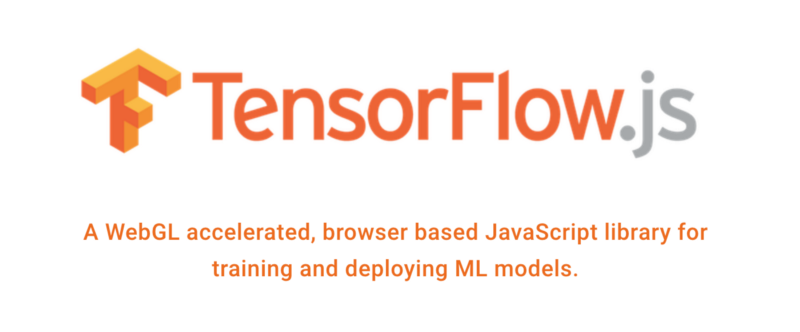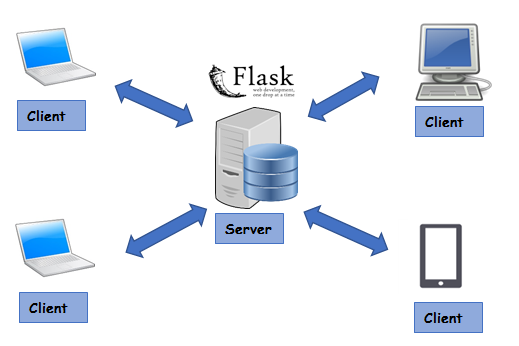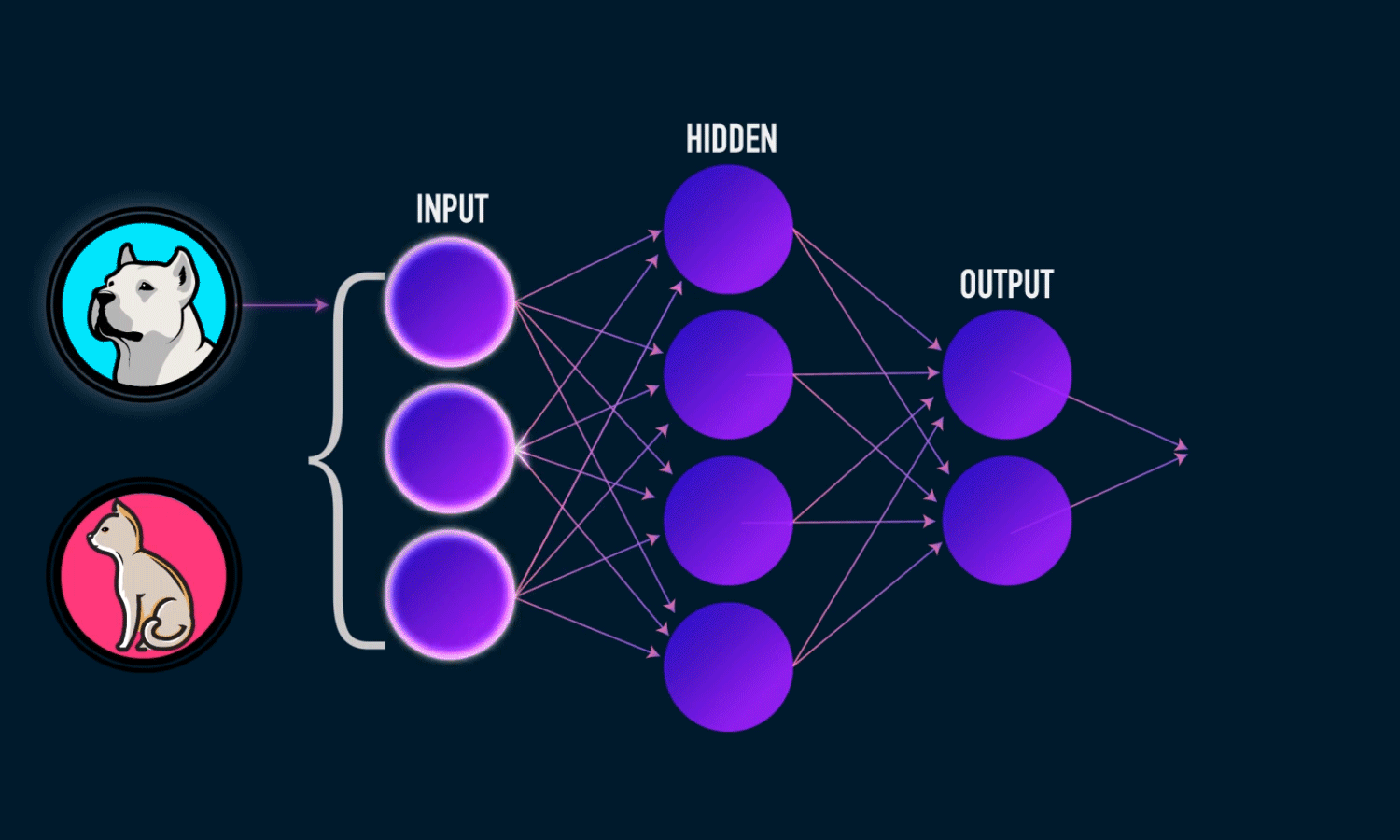by ADL

# Get to know TensorFlow.js in 7 minutes

#### And learn how you can run ML/DL models directly in the browserPhoto by Irvan Smith on Unsplash

An increasing number of developers are using TensorFlow in their machine learning projects. In March this year, the TensorFlow team at Google announced the arrival of the much-awaited JavaScript framework, TensorFlow.js (which was previously called DeepLearn.js).Image Source : Tensorflow.js Website

Now developers can build lightweight models and run them in the browser using JavaScript. Let’s understand what the need was for the development of this framework.

#### History

Before going to TensorFlow.js, I would like to start off with TensorFlow.

TensorFlow was developed in 2011 at Google as their propitiatory library for Machine learning/Deep learning applications at Google. This library was open sourced in 2015 under the Apache License.

TensorFlow is built in C++, which enables the code to execute at a very low level. TensorFlow has bindings to different language like Python, R, & Java. This enables TensorFlow to be used in these languages.

So, the obvious question is: what about JavaScript?

Conventionally, in JavaScript, ML/DL was performed by using an API. An API was made using some framework, and the model was deployed at the server. The client sent a request using JavaScript to get results from the server.Client Server Architecture

In 2017, a project called Deeplearn.js appeared, which aimed to enable ML/DL in JavaScript, without the API hassle.

But there were questions about speed. It was very well known that JavaScript code could not run on GPU. To solve this problem, WebGL was introduced. This is a browser interface to OpenGL. WebGL enabled the execution of JavaScript code on GPU.

In March 2018, the DeepLearn.js team got merged into the TensorFlow Team at Google and was renamed TensorFlow.js.

Watch the below video for further details:

### TensorFlow.js

Tensorflow.js provides two things:

• The CoreAPI, which deals with the low level code
• LayerAPI is built over the CoreAPI, and makes our lives easier by increasing the level of abstraction.

#### Getting Started

There are two main ways to get TensorFlow.js in your project:

#### 1. via <script> Tag

Add the following code to an HTML file:

``<html>  <head>    <!-- Load TensorFlow.js -->    <script src="https://cdn.jsdelivr.net/npm/@tensorflow/[email protected]"> </script>  </head>``
``<body>      Hello  </body></html>``

#### 2. via NPM

Add TensorFlow.js to your project using yarn or npm.

``yarn add @tensorflow/tfjs``
``npm install @tensorflow/tfjs``

In your main js file:

``import * as tf from '@tensorflow/tfjs';``

### CoreAPI

#### 1. Tensors

So, what is a Tensor ?

• A scalar is a single number. For example, x = 1
• A vector is an array of numbers. For example, x=[1,2]
• A matrix is a 2-D array
([[1, 2],
[3, 4],
[5, 6]])
• A tensor is a n-dimensional array with n>2

TensorFlow.js has utility functions for common cases like Scalar, 1D, 2D, 3D and 4D tensors, as well a number of functions to initialize tensors in ways useful for machine learning.

#### Code Examples

tf.tensor():

``// Pass an array of values to create a vector.tf.tensor([1, 2, 3, 4]).print();``

tf.scalar():

``tf.scalar(3.14).print();``

And so on…

Watch the Below Video to get a deep insight into Tensors in TensorFlow.js:

#### 2. Variables & Operations

Tensors are immutable data structures. That means their values can’t be changed once they are set.

However, `tf.variable()` is introduced in TensorFlow.js. The real use case for `tf.variable()` is when we need to change the data frequently, such as when adjusting model weights in Machine Learning.

Code sample:

``const x = tf.variable(tf.tensor([1, 2, 3]));x.assign(tf.tensor([4, 5, 6]));x.print();``

#### Operations

There are various operations in TensorFlow.js. In order to perform mathematical computation on Tensors, we use operations. Tensors are immutable, so all operations always return new Tensors and never modify input Tensors. So` tf.variable()` can be used in order to save memory.

Let’s look into some operations:

tf.add() — Adds two tf.Tensors element-wise

``const a = tf.tensor1d([1, 2, 3, 4]);const b = tf.tensor1d([10, 20, 30, 40]);a.add(b).print();  // or tf.add(a, b)``

There are many operations in TensorFlow.js. You can check the documentation for other operations. I will demonstrate one more operation here: tf.matmul()

tf.matmul() — Computes the dot product of two matrices, A * B.

``const a = tf.tensor2d([1, 2], [1, 2]);const b = tf.tensor2d([1, 2, 3, 4], [2, 2]);``
``a.matMul(b).print();  // or tf.matMul(a, b)``

Watch the below video for deep insight into Variable and Operations:

#### 3. Memory Management

Memory management is the key in Machine Learning/Deep Learning tasks, because they are generally computationally expensive.

TensorFlow.js provides two major ways to manage memory:

1. tf.dispose()
2. tf.tidy()

They both typically do the same thing, but they do it in different ways.

#### tf.tidy()

This executes the provided function `fn` and after it is executed, cleans up all intermediate tensors allocated by `fn` except those returned by `fn`.

`tf.tidy()` helps avoid memory leaks. In general, it wraps calls to operations in `tf.tidy()` for automatic memory cleanup.

Code example:

``const y = tf.tidy(() => {   // aa, b, and two will be cleaned up when the tidy ends.   const two= tf.scalar(2);   const aa = tf.scalar(2);   const b = aa.square();   console.log('numTensors (in tidy): ' + tf.memory().numTensors);   // The value returned inside the tidy function will return   // through the tidy, in this case to the variable y.   return b.add(two);});console.log('numTensors (outside tidy): ' + tf.memory().numTensors);y.print();``

#### tf.dispose()

Disposes any tf.Tensors found within the mentioned object.

Code example:

``const two= tf.scalar(2);``
``two.dispose()``

### LayersAPI

Layers are the primary building block for constructing a ML/DL Model. Each layer will typically perform some computation to transform its input to its output. Under the hood, every layer uses the CoreAPI of Tensorflow.js.

Layers will automatically take care of creating and initializing the various internal variables/weights they need to function. So, basically it makes life easier by increasing the level of abstraction.

We will make a simple example feed forward network using the LayerAPI. The Feed Forward network we will build is as below:Image is my own

#### Code:

Index.html

``<html><head><title></title><script src=”https://cdn.jsdelivr.net/npm/@tensorflow/[email protected]"> </script><script src=”main.js” type=”text/javascript”></script>``
``</head>``
``<body>Tensorflow JS Demo``
``</body></html>``

main.js

``const model = tf.sequential();``
``//config for layerconst config_hidden = {  inputShape:,  activation:'sigmoid',  units:4}const config_output={  units:2,  activation:'sigmoid'}``
``//defining the hidden and output layerconst hidden = tf.layers.dense(config_hidden);const output = tf.layers.dense(config_output);``
``//adding layers to modelmodel.add(hidden);model.add(output);``
``//define an optimizerconst optimize=tf.train.sgd(0.1);``
``//config for modelconst config={optimizer:optimize,loss:'meanSquaredError'}``
``//compiling the modelmodel.compile(config);``
``console.log('Model Successfully Compiled');``
``//Dummy training dataconst x_train = tf.tensor([  [0.1,0.5,0.1],  [0.9,0.3,0.4],  [0.4,0.5,0.5],  [0.7,0.1,0.9]])``
``//Dummy training labelsconst y_train = tf.tensor([  [0.2,0.8],  [0.9,0.10],  [0.4,0.6],  [0.5,0.5]])``
``//Dummy testing dataconst x_test = tf.tensor([  [0.9,0.1,0.5]])``
``train_data().then(function(){  console.log('Training is Complete');  console.log('Predictions :');  model.predict(x_test).print();})``
``async function train_data(){  for(let i=0;i<10;i++){  const res = await model.fit(x_train,y_train,epoch=1000,batch_size=10);   console.log(res.history.loss);  }}``

Output:

Watch the below videos for deep insight and code explanation:

I understand that this is a small overview on the Tensorflow.js Library. I feel this can be a starting point before you read the documentation and go through some real world applications.

I will be posting real world examples using TensorFlow.js as below:

More Real world examples coming soon…Stay Tuned

### My take on this

This is excellent for coders who are familiar with JavaScript and are trying to find their way in the ML/DL world!

It makes things a lot simpler for people coming from a non-ML/DL background, but who are looking to understand this field. The use cases for this are many, and I personally think it’s something we need at the moment.

In my next article and video, I will talk about ML5 which is built over TensorFlow.js. ML5 is built at New York University and is under active development.

What do you think about TensorFlow.js? Let me know in the comments section below. If you like this article, you would also like my Videos on Youtube.

If you liked my article, please click the ? below And follow me on Medium & :

If you have any questions, please let me know in a comment below or Twitter.